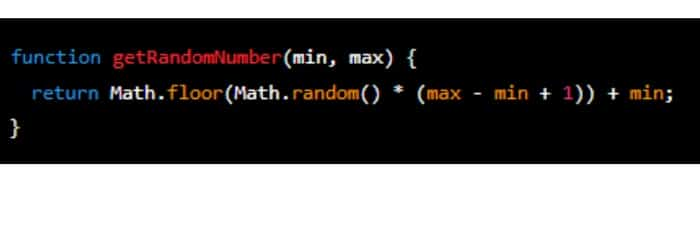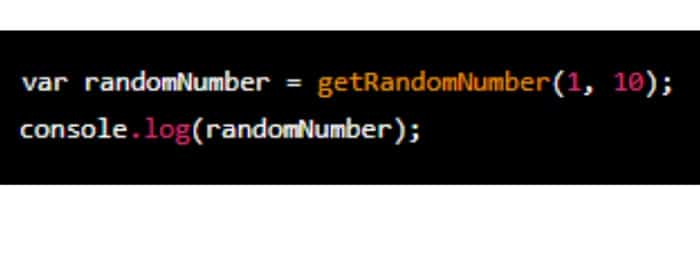# Random Number Generator Using JavaScript: Practical GuideRandom number generation is crucial to many applications, especially those in the gaming and gambling industries. In these applications, it is essential to generate random numbers that are unpredictable and unbiased. This is why random number generators find their use to shuffle cards in a deck, roll dice in a game, or choose a lucky winner in a contest. This article explains how to make a random number generator in JavaScript.JavaScript is one of the leading technologies of the World Wide Web, with HTML and CSS as ActionListener. It provides built-in functions for random number generation, making creating a random number generator easy.

## Generating Random Numbers In JavaScript

The Math.random() function is the built-in function in JavaScript for generating random numbers. Math.random() function returns a random number in 0 (inclusive) to 1 (exclusive). To generate a random number within a specific range, we can multiply the result of Math.random() by the range and then use the floor() function to round down to the nearest integer.

Here is an example of how to generate a random number between 1 and 10 in javascript:In the example above, we multiplied Math.random() by 10 to get a number between 0 and 10, and we added 1 to get a number between 1 and 10. Finally, we used the floor() function that always rounds down and returns the largest integer less than or equal to a given number.

## Creating A Random Number Generator Function

To make our random number generator more reusable, we can create a function that accepts a range and returns a random number within that range. Here is an example of a random number generator function:In the example above, the getRandomNumber() function accepts two parameters, min and max, representing the range’s minimum and maximum values. The function calculates a random number within the field by subtracting the minimum from the maximum to get the size of the range, then multiplying Math.random() by the size of the range, and finally adding the minimum to the result.

## Using The Random Number Generator Function

To use the random number generator function, we call it and pass in the minimum and maximum values of the range. Here is an example:In the example above, we called the getRandomNumber() function by passing the minimum and maximum values as 1 and 10. The function then returns a random number between 1 and 10, which we logged into the console.

## FAQs

### What is the most common method for generating random numbers in JavaScript?

The most widely used method in JavaScript for generating random numbers is Math.random(). It gives back an unexpected floating-point result between 0 and 1, inclusive. You can modify the outcome in line with your needs to produce integers within a particular range.

### Is Math.random() a secure random number generator regarding cryptography?

No, Math.random() is not secure regarding cryptography. It should not be used in applications that require security because it utilizes a pseudo-random number generator (PRNG). For secure random number generation, the crypto.getRandomValues() method is available in modern browsers and Node.js environments.

### What's the difference between Math.random() and crypto.getRandomValues()?

Math.random() generates pseudo-random numbers, meaning that if you know the initial state (seed), you can predict the sequence of numbers it produces. On the other hand, crypto.getRandomValues() provides cryptographically secure random numbers, making it suitable for cryptographic operations and other security-sensitive tasks.

### Can Math.random() produce random integers within a specific range?

You can generate random integers within a specified range using Math.random(). You can use Math.floor(Math.random() * (max-min + 1)) + min to achieve this.

### Are there any external libraries for advanced random number generation in JavaScript?

Yes, external libraries are available that provide more advanced random number generation techniques or offer additional features. One popular library for this purpose is lodash, which includes functions like _.random().

### Why is it essential to use a cryptographically secure random number generator for sensitive tasks?

Cryptographically secure random number generators ensure the generated numbers are not predictable, making them suitable for encryption, fast key generation, password hashing, and other cryptographic operations. Using non-secure random number generators could expose vulnerabilities and compromise security.

## Conclusion

Random number generators are a helpful tool in many applications, and JavaScript provides a built-in function for generating random numbers. Creating a random number generator function can make our code more reusable and maintainable. With a few lines of code, you can easily create a random number generator in JavaScript. You can also use it in various applications.